Pólya distribution

The probability distribution of a random variable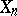taking non-negative integer values,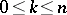, given by the formula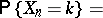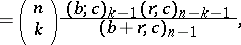whereand the integers,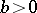,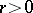, and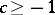are parameters; or, when, give by the equivalent formula(2)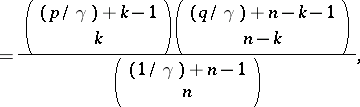where the integer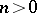, the real numbers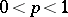,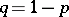, and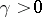are parameters. The relation between

and (2) is given byThe mathematical expectation and variance of the Pólya distribution are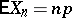and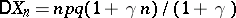, respectively. Special cases of the Pólya distribution are:has a binomial distribution with parametersandfor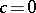;has a hypergeometric distribution forwith parameters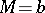,and. For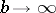andsuch thatis constant and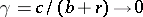, the distribution tends to the binomial distribution with parametersand.

The distribution was considered by G. Pólya (1923) in connection with the so-called Pólya urn scheme. From an urn containingblack andred balls one makes a selection with replacement, subject to the condition that each extracted ball is returned to the urn together withballs of the same colour. Ifis the total number of black balls at the-th trial, the distribution ofis given by

or (2). The sequence,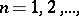is a discrete Markov process, where the states are defined by the numbers of black balls in the sample at time, while the conditional probability of transition from a stateat timeto a state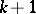at timeequals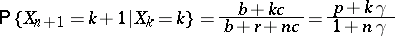(this depends on).

By passing to the limit from the Pólya urn scheme one obtains the Pólya process, which is an inhomogeneous Markov process in continuous time and belongs to the class of "pure multiplication" processes. Under the condition that there is only one extraction of a ball in an infinitesimal time, one obtains the conditional limit probability of transition from the stateto state theduring the time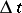forwhen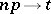and, asOn transition from the Pólya urn scheme to the Pólya process one obtains an important limit form for the Pólya distribution. That is, the probabilityof remaining in the stateat timeis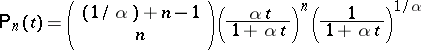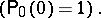This limit distribution is the negative binomial distribution with parameters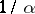and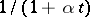(the corresponding mathematical expectation is, while the variance is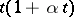).

The urn model and the Pólya process, in which the Pólya distribution and the limit form of it arise, are models with an after effect (extracting a ball of a particular colour from the urn increases the probability of extracting a ball of the same colour in a subsequent trial).

Astends to zero, the Pólya process goes over to a Poisson process, while the Pólya distribution forhas as limit the Poisson distribution with parameter.